Geometry

# Tangent - Subtended Arc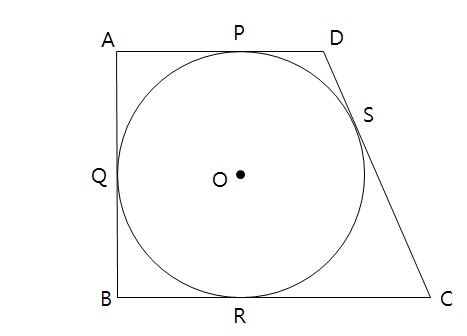Circle $O$ with radius $2$ is inscribed in a trapezoid $ABCD$ such that $\overline{AD} \parallel \overline{BC}$ and $\lvert \overline{CD} \rvert = 8.$ If $\angle{A} = \angle{B} = 90^{\circ},$ what is the area of $ABCD?$

Note: The above diagram is not drawn to scale.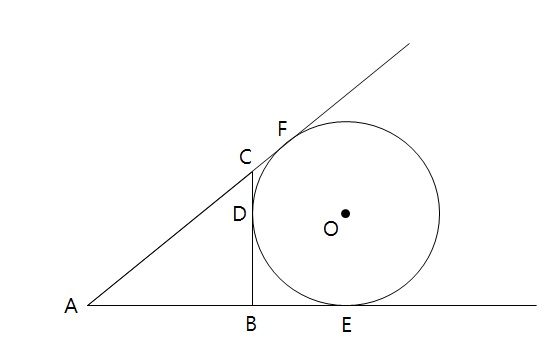In the above diagram, $\overline{BC}, \overline{AE}$ and $\overline{AF}$ are tangent to circle $O$ at $D, E$ and $F,$ respectively. If $\lvert \overline{AC} \rvert = 25, \lvert \overline{AB} \rvert = 20, \lvert \overline{BC} \rvert = 15,$ what is the area of quadrilateral $CDOF?$

Note: The above diagram is not drawn to scale.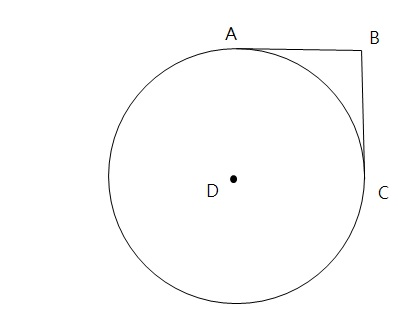In the above diagram, $\overline{AB}$ and $\overline{CB}$ are both tangent to circle $D$ and are perpendicular to each other. If the length of $\overline{AB}$ is $8,$ what is the area of the quadrilateral $ABCD?$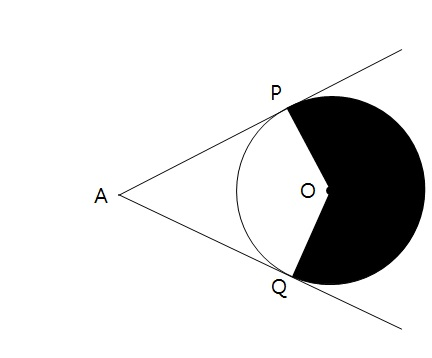In the above diagram, $\overline{AP}$ and $\overline{AQ}$ are tangent to circle $O$ at $P$ and $Q,$ respectively. If the length of $\overline{AP}$ is $\lvert \overline{AP} \rvert = 13$ and $\angle{PAQ} = 60^{\circ}$, what is the area of the shaded region?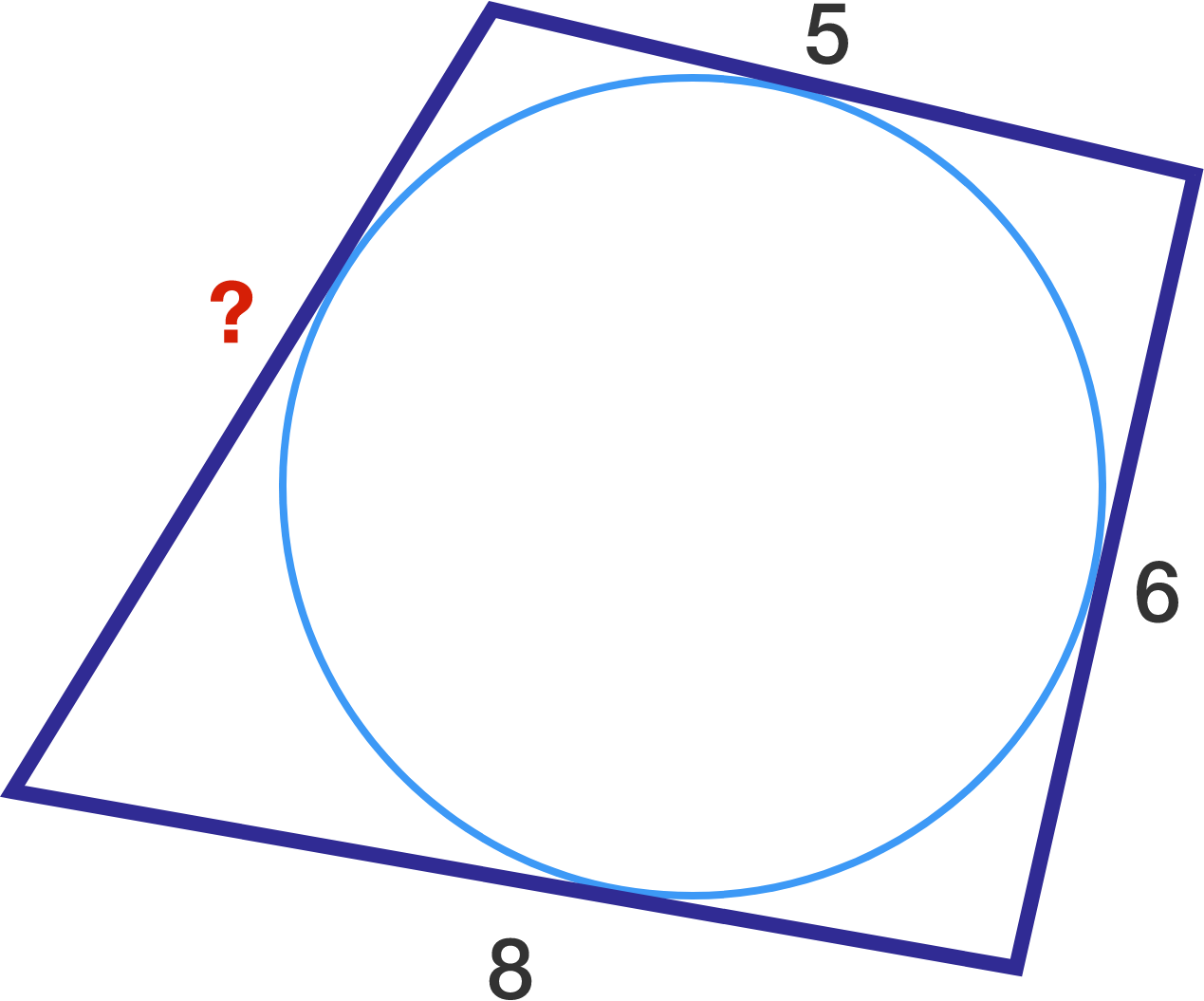Given the quadrilateral and inscribed circle, what is the missing side length?

×